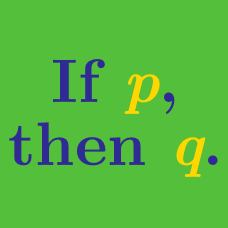Everyday Math

# Counter-Examples

If $n$ is a prime integer less than or equal to $10,$ then $n^2+2$ is prime.

How many counter-examples are there to the above claim?

(A) $\ 0$
(B) $\ 1$
(C) $\ 2$
(D) $\ 3$
(E) $\ 4$

A real number $x$ is called nearly-even, if there is an even integer $n$ such that

$| x - n | < 0.5.$

If $X$ is a nearly-even number, which of the following statements must be true?

$\begin{array}{r r l} & \text{I.}\ & X\ \text{is an even integer.}\\ & \text{II.}\ & 6X\ \text{is an integer.}\\ & \text{III.}\ & \text{The integer part of}\ 6X\ \text{is even.}\\ \end{array}$

(A) $\ \$ II only
(B) $\ \$ I and II only
(C) $\ \$ I and III only
(D) $\ \$ II and III only
(E) $\ \$ None of the statements

If $n$ is prime, then $2n+1$ is also prime.

Which of the following is a counter-example of the above claim?

(A) $\ 1$
(B) $\ 2$
(C) $\ 3$
(D) $\ 5$
(E) $\ 7$

A number is called 4-average if it is the average of four positive integers. If $k$ is a 4-average number, which of the following statements is true?

$\begin{array}{r r l} &\text{I.}\ & k\ \text{is an integer.}\\ &\text{II.}\ & 4k\ \text{is an integer.}\\ &\text{III.}\ & k\ \text{is positive.}\\ \end{array}$

(A)$\ \$ II only
(B)$\ \$ III only
(C)$\ \$ II and III only
(D)$\ \$ I, II, and III
(E)$\ \$ None of the statements

If $f\left(f(x)\right)=x,$ then $f(x)=x.$

Which of the following is a counter-example of the above claim?

(A) $\ f(x)=1, x \in \mathbb{R}$
(B) $\ f(x)=x, x \in \mathbb{R}$
(C) $\ f(x)=\frac{1}{x}, x > 0$
(D) $\ f(x)=x^2, x > 0$
(E) $\ f(x)=\sqrt{x}, x > 0$

×